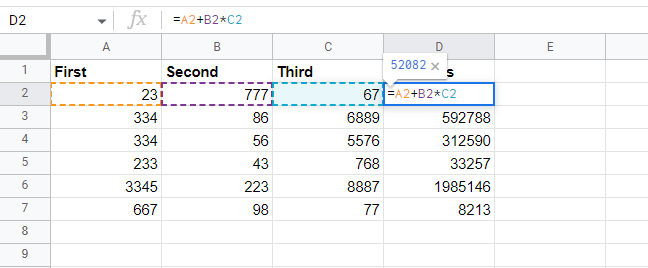# How to modify an existing formula in Google Sheets Templates?

Published June 16, 2022

There are many reasons why you might want to make changes to an existing formula in Google Sheets; either you want to change cell references, the mathematical operators used, make a specific cell reference constant or even change the order of operations. In other words, you might go in and fix a typo in a formula, for instance. Check out the steps below to edit an existing formula:

Steps to editing an existing formula in Google Sheets

Step 1: Double click on the cell containing the formula you would like to modify. `By doing so, you can view the formula used in the mathematical operation. In this case, the formula used is =A2+B2*C2Step 2: Depending on your needs, you can now modify your operator and cell references or make one cell reference constant. Here, we will add one more mathematical operator, which will change the order of operations of the formula. Our new formula will be: =A2/B2+B2*C2Step 3: Next, we need to edit the formulas in the rest of column D. To do so, click the fill handle and drag the handle down the column to apply the edited formula to the cells below. From D2 to D7, we'll copy and paste the formula.Step 4: When you release your mouse, the values from the new formula will be displayed in column D.Step 5: Verify that the new formula has been applied to all cells by double-clicking on any cell. The new formula has been implemented in all cells.Conclusion

In this article, we have learned how to modify the existing formula to fit our needs. In the new formula, the cell values are recalculated and displayed

 Article Contributed By :50 Views